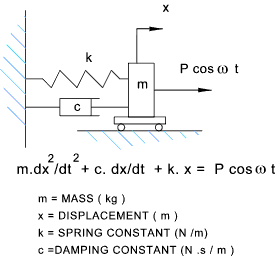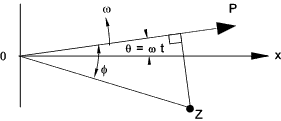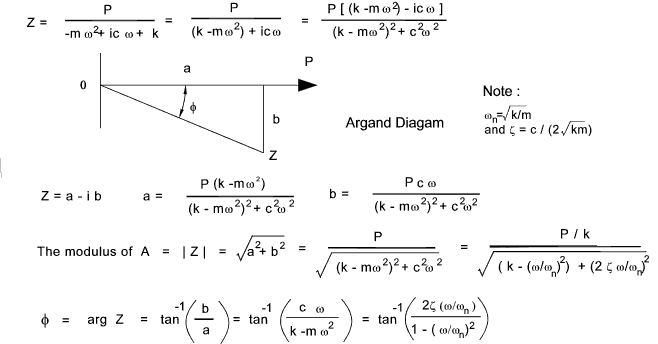Vibrations Index Page

Forced Vibrations
 Introduction These initial notes relate to single degree of freedom systems under the influence of periodic excitation forces i.e.the force is sinusoidal in nature.   If the solution of this general case is known then, in principle, other more complicated period forces can be determined by superimposing the effects of as many sinusoidal components as required.  Other force types e.g. rotating unbalanced forces will be addressed later.   Note: This page includes use of complex numbers.Information on manupulation of complex numbers is found on webpage Complex Numbers Forced VibrationThe equation of motion for the above system is m . dx2 /dt 2 + c . dx /dt + k . x = P cos ω t The general solution of the equation is the sum of two parts 1)The complementary function which is the general solution assuming the right hand side set at zero 2)The particular solution which depends on the form of the right hand side of the equation. Generally the systems under consideration are linear and the Superposition Principle applies allowing both functions to be determined separately and then added. x = xc + xp The amplitude of the complementary solution decreases with time and is therefore a transient part of the general solution.  Notes on solving the complementary solution are provided on webpage Free vibrations The particular part remains over time and is really a steady state solution.  The notes belw relate to solution of the steady state solution. As the right hand of the equation of motion is sinusoidal a convenient solution is based on the relationship (Euler formula ) e iθ = cosθ + i sin θ putting ω t in place of θ e iω t = cosω t + i sin ω t Note: cos ω t is the real component of ei ω t. A particular solution of the equation of motion is obtained by use of the formula x = B cos ω t + C sin ω t.   Another form of this equation is x = A cos (ω t - φ ) Substitution of the above into the Euler formula results in the equation xp = Real( A e i (ω t - φ)) = Real ( A e -i φ e i ω t ) Next substitute Z for the complex constant A e -iφ   The modulus of Z is the amplitude of the steady state solution .   The argument of Z is φ, the phase of the steady state solution. An argand diagram of this equation is shown below.note: Referring to the initial equation a)  xp = Real ( Z e i ω t ) b)  dx/dt = Real ( i ω t Z e iω t ) c)  dx2/dt2 = Real ( i 2 ω 2 Z e iω t ) Substituting these values into the original equation Real [ ( -m ω2 + i c ω + k ) Z e i ω t ] = Real ( P e i ω t ) The solution for x is provided if P = ( -m ω2 + i c ω + k ) ZThe steady state solution is therefore: x = A cos ( ω t - φ )

 Links on Forced Vibration Damped Forced Vibrations Tool...Java applet providing visual display or forced vibrations E-A-R speciality Composites ...site includes useful papers on vibration Forced Vibrations...Tool for experimenting with forced vibrations Forced Vibrations...Useful lecture notes Basic Theory of Vibrationss...Chapter tow of a text boom by RE Blake
Vibrations Index Page